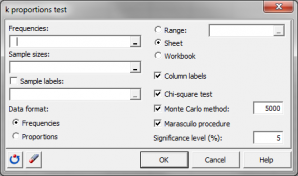# k proportions test

k-proportions tests compare several observed proportions to one another. Run k-proportions tests in Excel using the XLSTAT add-on statistical software.## What is a k-proportions comparison test

This type of parametric test is used to determine k (k>2) proportions can be considered as equal (null hypothesis H0) or if at least two proportions are significantly different (alternative hypothesis Ha).

## Tests used for the comparison of k proportions

XLSTAT offers three different approaches to compare k proportions.

### Chi-square test

This test is identical to that used for the contingency tables.

### Monte Carlo method

The Monte Carlo method is used to calculate a distribution of the Chi² distance based on simulations with the constraint of complying with the total number of observations for the k groups. This results in an empirical distribution which gives a more reliable critical value (on condition that the number of simulations is large) than that given by the Chi-square theoretical distribution which corresponds to the asymptotic case.

## Marascuilo procedure for multiple comparisons of proportions

It is advised to use the Marascuilo procedure only if the Chi-square test or the equivalent test based on Monte Carlo simulations reject H0. The Marascuilo procedure compares all pairs of proportions, which enables the proportions possibly responsible for rejecting H0 to be identified.### analyze your data with xlstat

14-day free trial

Included in

Related features